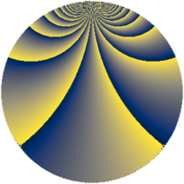# Properties

 Label 1152.3.jLevel $1152$ Weight $3$ Character orbit 1152.j Rep. character $\chi_{1152}(161,\cdot)$ Character field $\Q(\zeta_{4})$ Dimension $64$ Newform subspaces $2$ Sturm bound $576$ Trace bound $19$

# Learn more

## Defining parameters

 Level: $$N$$ $$=$$ $$1152 = 2^{7} \cdot 3^{2}$$ Weight: $$k$$ $$=$$ $$3$$ Character orbit: $$[\chi]$$ $$=$$ 1152.j (of order $$4$$ and degree $$2$$) Character conductor: $$\operatorname{cond}(\chi)$$ $$=$$ $$48$$ Character field: $$\Q(i)$$ Newform subspaces: $$2$$ Sturm bound: $$576$$ Trace bound: $$19$$

## Dimensions

The following table gives the dimensions of various subspaces of $$M_{3}(1152, [\chi])$$.

Total New Old
Modular forms 832 64 768
Cusp forms 704 64 640
Eisenstein series 128 0 128

## Trace form

 $$64q + O(q^{10})$$ $$64q - 448q^{49} - 128q^{61} - 640q^{85} + O(q^{100})$$

## Decomposition of $$S_{3}^{\mathrm{new}}(1152, [\chi])$$ into newform subspaces

Label Dim. $$A$$ Field CM Traces $q$-expansion
$$a_2$$ $$a_3$$ $$a_5$$ $$a_7$$
1152.3.j.a $$32$$ $$31.390$$ None $$0$$ $$0$$ $$0$$ $$0$$
1152.3.j.b $$32$$ $$31.390$$ None $$0$$ $$0$$ $$0$$ $$0$$

## Decomposition of $$S_{3}^{\mathrm{old}}(1152, [\chi])$$ into lower level spaces

$$S_{3}^{\mathrm{old}}(1152, [\chi]) \cong$$ $$S_{3}^{\mathrm{new}}(48, [\chi])$$$$^{\oplus 8}$$$$\oplus$$$$S_{3}^{\mathrm{new}}(144, [\chi])$$$$^{\oplus 4}$$$$\oplus$$$$S_{3}^{\mathrm{new}}(192, [\chi])$$$$^{\oplus 4}$$$$\oplus$$$$S_{3}^{\mathrm{new}}(384, [\chi])$$$$^{\oplus 2}$$$$\oplus$$$$S_{3}^{\mathrm{new}}(576, [\chi])$$$$^{\oplus 2}$$